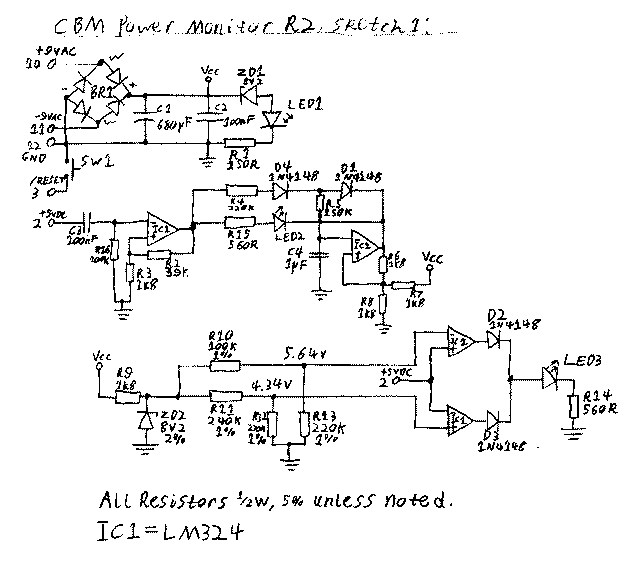# Computer Nerd Kev

### Commodore Power Monitor Circuit DescriptionThe schematic for the Power Monitor.

The circuit is divided into three sections for the different warning functions:

9VAC

This part of the circuit also powers the LM324 Quad Op-Amp and shows the RESET switch for the computer, which simply connects the "/RESET" line to GND when pressed. A Bridge Rectifier, as well as C1 and C2, provide the DC voltage for the circuit, around 11.3V. Following this, an 8.2V Zener Diode (ZD1) is placed in series with the 9VAC LED (which will have a voltage drop of around 2V across itself), therefore if the 9VAC line drops about 1V peak (equivalet to 8.3VAC) the LED will not light or be very dim. R1 limits the current through the LED.

RIPPLE

The 5VDC line from the computer is applied to a non-inverting input of the LM324 Quad Op-Amp via DC blocking capacitor, C3. R2 and R3 set the gain of the Amp to around 33. The output is fed to LED2 (RIPPLE) via current limiting resistor R15, and to C4 and the Inverting input of another Amp (whose output is High) via R4, D4 and R5. When the amplified ripple voltage is above the threshold, C4 will charge past the 2/3Vcc voltage set by the voltage divider at the Non-Inverting Input consisting of R6, R7, R8, and the output will swing Low, allowing the LED to light. C4 now discharges to the Op-Amp output via R5, D1, and when it is below the 1/3Vcc voltage at the Non-Inverting Input, the output will again swing High, turning off the LED and continuing the oscillation until the 5VDC ripple voltage decreases.

5VDC

The 11.3V DC circuit supply is regulated by an 8.2V Zener Diode (ZD2) and current limiting resistor, R9. Two voltage dividers are used for High and Low voltages, a three resistor voltage divider was not used to minimise the effects of component variation. These voltages feed into two Op-Amps arranged as a Window Comparator to turn on LED3 (5VDC) if the 5VDC voltage rises above or below the references.